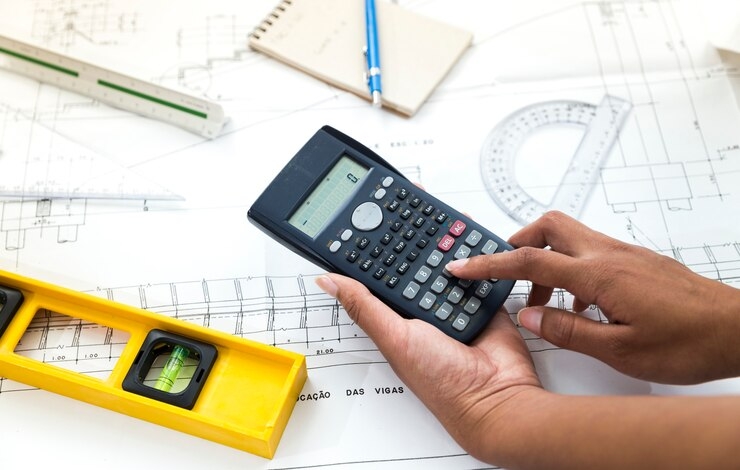# This is How Civil Engineers Perform Area Calculations!0

What is this blog post about?

# This is How Civil Engineers Perform Area Calculations!Image by pressfoto on Freepik

It would be no wrong to say that civil engineering is another name for the bridge that connects the physical worlds to the architectural networks. And as every civil engineer knows better that area is one of the basic and most important parameters that decides how much space a certain land piece is occupying.

This calculation can either be a whole number or a fraction that needs to be reduced if you need to get accurate area estimations. The calculation issue arises when engineers have to draw a map of a land. This is where the chances of negligence are not ignorable.

Gone are the days when it was a daunting chore to calculate area in different units of measurement. Nowadays, engineers use online feet and inches calculators for this purpose. After they get complicated area calculations in the form of fractions, they just need to enter values in this tool and get straightforward results in seconds.

The calculator basically performs arithmetic operations on fractions related to feet and inches. If you are studying civil engineering, you must not ignore the importance of this online tool.

## How Feet and Inches Calculator Function?

Most of the tools work in the same way. It is up to you how you input values. But a generic review of different online calculators has been done by us. This is why we have summarized the guide so you may not feel trouble while adding or subtracting feet and inches:
• Open the tool you trust most
• Enter the feet and inches for the first measurement you made
• Do the same for the second measurement
• Now select the arithmetic operation you want to perform
• And this is how you are done

## Foundational Understanding of Area Calculations:Image by Freepik

It is essential to have a fundamental understanding of area calculation before going more deeply into the procedures. Finding out how much space is contained within a certain border can be seen as another way of expressing the concept of determining an area.

The calculation appears to be very straightforward for fundamental geometric shapes like squares and rectangles. However, engineering projects that are really carried out in the real world frequently involve a combination of simple and complicated geometries, which calls for a more complex approach.

This is where the feet and inches calculator can really assist. The tool provides an edge to perform instant simplification of complicated area calculations done in units of feet and inches.

You can get a step by step calculations as well that lets you understand how the results have been driven. An excellent approach if you utilize it organically.

## Important Feet and Inches Calculations:

Below we have compiled some basic equations that might help you perform area calculations:

### Rectangle Area:

𝐴𝑟𝑒𝑎 = 𝐿𝑒𝑛𝑔𝑡ℎ (𝑖𝑛 𝑓𝑒𝑒𝑡) 𝑥 𝑊𝑖𝑑𝑡ℎ (𝑖𝑛 𝑓𝑒𝑒𝑡)

### Triangle Area:

𝐴𝑟𝑒𝑎 = 0. 5 𝑥 𝐵𝑎𝑠𝑒 (𝑖𝑛 𝑓𝑒𝑒𝑡) 𝑥 𝐻𝑒𝑖𝑔ℎ𝑡 (𝑖𝑛 𝑓𝑒𝑒𝑡)

### Circle Area:

𝐴𝑟𝑒𝑎 = π 𝑥 𝑅𝑎𝑑𝑖𝑢𝑠^2 𝑊ℎ𝑒𝑟𝑒 π (𝑝𝑖)

After you are done with your area calculations, you can reduce them to the most simplified form with the feet and inches calculator. Get steps involved so that you may get to know how to get it done manually.

## Application in the Real World:

Consider a piece of land with the shape of a trapezoid. This shape is typical in civil engineering projects. The average of the two parallel sides is multiplied by the height to determine the area.

Before arriving at the final area, a sequence of conversions between feet and inches would be required if one side is 10 feet 6 inches long and the other is 15 feet 3 inches, with a height of 7 feet 8 inches.

This is where civil engineers might face hurdles in calculations. But feet and inches calculator can certainly help them to overcome complicated calculations and get answers in seconds.

## Last Words:

Civil engineering is one of the major engineering disciplines that is based upon very simple concepts. But when dealing with complicated measurements, engineers might face difficulty in simplifying the results. This creates a wall between your thoughts and a good map design as well. This is why as a civil engineer, you must comprehend the basics calculations like area, volume, etc. these basics will strong your thinking and critical approach.